# 1.3: 整数

•• OpenStax
• OpenStax
$$\newcommand{\vecs}{\overset { \rightharpoonup} {\mathbf{#1}} }$$ $$\newcommand{\vecd}{\overset{-\!-\!\rightharpoonup}{\vphantom{a}\smash {#1}}}$$$$\newcommand{\id}{\mathrm{id}}$$ $$\newcommand{\Span}{\mathrm{span}}$$ $$\newcommand{\kernel}{\mathrm{null}\,}$$ $$\newcommand{\range}{\mathrm{range}\,}$$ $$\newcommand{\RealPart}{\mathrm{Re}}$$ $$\newcommand{\ImaginaryPart}{\mathrm{Im}}$$ $$\newcommand{\Argument}{\mathrm{Arg}}$$ $$\newcommand{\norm}{\| #1 \|}$$ $$\newcommand{\inner}{\langle #1, #2 \rangle}$$ $$\newcommand{\Span}{\mathrm{span}}$$ $$\newcommand{\id}{\mathrm{id}}$$ $$\newcommand{\Span}{\mathrm{span}}$$ $$\newcommand{\kernel}{\mathrm{null}\,}$$ $$\newcommand{\range}{\mathrm{range}\,}$$ $$\newcommand{\RealPart}{\mathrm{Re}}$$ $$\newcommand{\ImaginaryPart}{\mathrm{Im}}$$ $$\newcommand{\Argument}{\mathrm{Arg}}$$ $$\newcommand{\norm}{\| #1 \|}$$ $$\newcommand{\inner}{\langle #1, #2 \rangle}$$ $$\newcommand{\Span}{\mathrm{span}}$$$$\newcommand{\AA}{\unicode[.8,0]{x212B}}$$

##### 摘要

• 使用绝对值简化表达式
• 加上和减去整数
• 整数的乘法和除法
• 使用整数简化表达式
• 使用整数计算变量表达式
• 将短语翻译成带整数的表达式
• 在应用程序中使用整数

## 使用绝对值简化表达式$$\PageIndex{1}$$数字线显示正数和负数的位置。

##### 对面$$\PageIndex{2}$$3 的反面是$$−3$$

##### 相反的符号

\begin{align} & -a \text{ means the opposite of the number }a \\ & \text{The notation} -a \text{ is read as “the opposite of }a \text{.”} \end{align}

##### 定义：绝对值

\begin{align} & -5 \text{ is } 5 \text{ units away from 0, so } |-5|=5. \\ & 5 \text{ is }5\text{ units away from 0, so }|5|=5. \end{align}图$$\PageIndex{3}$$：数字 5 和 −5 与 0 相距 5 个单位。

##### 示例$$\PageIndex{1}$$

$$=$$为以下每对数字填写$$<,\,>,$$或：

1. $$\mathrm{|−5|}\_\_\mathrm{−|−5|}\_\_\mathrm{−|5|}$$
2. $$\text{8__−|−8|}$$
3. $$\text{−9__−|−9|}$$
4. (\ text {− (−16) __|−16|}\)。

$$\begin{array}{lrcc} { \text{ } \\ \text{Simplify.} \\ \text{Order.} \\ \text{ } } & {|−5| \\ 5 \\ 5 \\ |−5|} & {\_\_ \\ \_\_ \\ > \\ >} & {−|−5| \\ −5 \\ −5 \\ −|−5|} \end{array}$$

b。

$$\begin{array}{llcc} { \text{ } \\ \text{Simplify.} \\ \text{Order.} \\ \text{ } } & {8 \\ 8 \\ 8 \\ 8} & {\_\_ \\ \_\_ \\ > \\ >} & {−|−8| \\ −8 \\ −8 \\ −|−8|} \end{array}$$

c。

$$\begin{array}{lrcc} { \text{ } \\ \text{Simplify.} \\ \text{Order.} \\ \text{ } } & {−9 \\ −9 \\ −9 \\ −9} & {\_\_ \\ \_\_ \\ = \\ =} & {−|−9| \\ −9 \\ −9 \\ −|−9|} \end{array}$$

d。

$$\begin{array}{lrcc} { \text{ } \\ \text{Simplify.} \\ \text{Order.} \\ \text{ } } & {−(−16) \\ 16 \\ 16 \\ −(−16)} & {\_\_ \\ \_\_ \\ = \\ =} & {−|−16| \\ 16 \\ 16 \\ |−16|} \end{array}$$

##### 示例$$\PageIndex{2}$$

$$=$$为以下每对数字填写$$<,\,>,$$或：

$$−9 \_\_−|−9|$$$$2 \_\_−|−2|$$$$−8 \_\_|−8|$$$$−(−9) \_\_|−9|.$$

$$>$$$$>$$$$<$$

$$=$$

##### 示例$$\PageIndex{3}$$

$$=$$为以下每对数字填写$$<,>,$$或：

1. $$7 \_\_ −|−7|$$
2. $$−(−10) \_ \_|−10|$$
3. $$|−4| \_\_ −|−4|$$
4. $$−1 \_\_ |−1|.$$

$$>$$$$=$$$$>$$

$$<$$

##### 分组符号

$\begin{array}{lclc} \text{Parentheses} & () & \text{Braces} & \{ \} \\ \text{Brackets} & [] & \text{Absolute value} & ||\end{array}$

##### 示例$$\PageIndex{4}$$

$$\begin{array}{lc} \text{} & 24−|19−3(6−2)| \\ \text{Work inside parentheses first:} & \text{} \\ \text{subtract 2 from 6.} & 24−|19−3(4)| \\ \text{Multiply 3(4).} & 24−|19−12| \\ \text{Subtract inside the absolute value bars.} & 24−|7| \\ \text{Take the absolute value.} & 24−7 \\ \text{Subtract.} & 17 \end{array}$$

16

9

## 加减整数

$\begin{array}{ll} \text{Counting numbers} & 1,2,3… \\ \text{Whole numbers} 0,1,2,3…. \end{array}$

##### 定义：整数

$…-3,-2,-1,0,1,2,3…,$$5+3 \; \; \; \; \; \; −5+(−3) \; \; \; \; \; \; −5+3 \; \; \; \; \; \; \; 5+(−3)$##### 示例$$\PageIndex{7}$$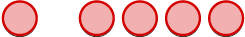1 个负面加上 4 个负面是 5 个负面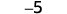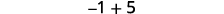有更多的正数，所以总和是正数。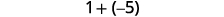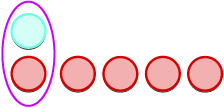还有更多的负数，所以总和是负数。##### 示例$$\PageIndex{8}$$

$$−6$$$$2$$$$−2$$

##### 示例$$\PageIndex{9}$$

$$−7$$$$3$$$$−3$$

$5−3 \; \; \; \; \; \; −5−(−3) \; \; \; \; \; \; −5−3 \; \; \; \; \; \; 5−(−3)$对第一个数字进行建模。我们现在添加所需的中性对。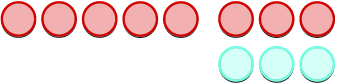我们删除以第二个数字建模的计数器数量。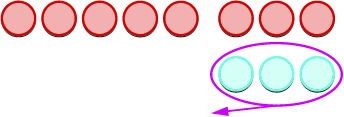数一下还剩下什么。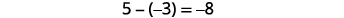##### 示例$$\PageIndex{10}$$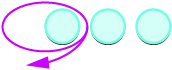从 3 个阳性中取出 1 个阳性，得到 2 个阳性。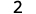从 3 个阴性中取出 1 个阳性，得到 2 个阴性。从添加的一对中性线中取出 1 个正数。从添加的一对中性线中取出 1 个负数。##### 示例$$\PageIndex{11}$$

$$2$$$$−2$$$$−10$$$$10$$

##### 示例$$\PageIndex{12}$$

$$3$$$$−3$$$$−11$$$$11$$

##### 定义：减法属性

$a−b=a+(−b)$

##### 示例$$\PageIndex{13}$$

$$\begin{array}{lccc} \text{} & 13−8 & \text{and} & 13+(−8) \\ \text{Subtract.} & 5 & \text{} & 5 \end{array}$$

$$\begin{array}{lccc} \text{} & −17−9 & \text{and} & −17+(−9) \\ \text{Subtract.} & −26 & \text{} & −26 \end{array}$$

$$\begin{array}{lccc} \text{} & 9−(−15) & \text{and} & 9+15 \\ \text{Subtract.} & 24 & \text{} & 24 \end{array}$$

$$\begin{array}{lccc} \text{} & −7−(−4) & \text{and} & −7+4 \\ \text{Subtract.} & −3 & \text{} & −3 \end{array}$$

##### 示例$$\PageIndex{14}$$

$$8,8$$$$−18,−18$$

$$19,19$$$$−4,−4$$

##### 示例$$\PageIndex{15}$$

$$8,8$$$$−22,−22$$

$$23,23$$$$3,3$$

##### 示例$$\PageIndex{16}$$

$$\begin{array}{lc} \text{} & 7−(−4−3)−9 \\ \text{Simplify inside the parentheses first.} & 7−(−7)−9 \\ \text{Subtract left to right.} & 14−9 \\ \text{Subtract.} & 5 \end{array}$$

3

13

## 乘以和除以整数$\begin{array}{ll} 5·3=15 & −5(3)=−15 \\ 5(−3)=−15 & (−5)(−3)=15 \end{array}$

$\text{signs are the } \textbf{same} \text{, the product is } \textbf{positive.} \\ \text{signs are } \textbf{different} \text{, the product is } \textbf{negative.}$

$\begin{array}{lclrccl} 5·3=15 & \text{so} & 15÷3=5 & \text{ } −5(3)=−15 & \text{so} & −15÷3=−5 \\ (−5)(−3)=15 & \text{so} & 15÷(−3)=−5 & \text{ } 5(−3)=−15 & \text{so} & −15÷(−3)=5 \end{array}$

• 两个积极因素 阳性
• 两张底片 阳性

• 正面和负面 负面的
• 负面和阳性 负面的

##### 示例$$\PageIndex{19}$$

$$\begin{array}{lc} \text{} & −100÷(−4) \\ \text{Divide, with signs that are} \\ \text{the same the quotient is positive.} & 25 \end{array}$$

$$\begin{array} {lc} \text{} & 7·6 \\ \text{Multiply, with same signs.} & 42 \end{array}$$

$$\begin{array} {lc} \text{} & 4(−8) \\ \text{Multiply, with different signs.} & −32 \end{array}$$

$$\begin{array}{lc} \text{} & −27÷3 \\ \text{Divide, with different signs,} \\ \text{the quotient is negative.} & −9 \end{array}$$

##### 示例$$\PageIndex{20}$$

ⓐ 23 ⓑ 60 ⓒ −63 ⓓ −9

ⓐ 39 ⓑ 39 ⓒ −28 ⓓ −7

##### 乘以 −1

$−1a=−a$

## 使用整数简化表达式

##### 示例$$\PageIndex{22}$$

$$\begin{array}{lc} \text{} & (−2)^4 \\ \text{Write in expanded form.} & (−2)(−2)(−2)(−2) \\ \text{Multiply.} & 4(−2)(−2) \\ \text{Multiply.} & −8(−2) \\ \text{Multiply.} & 16 \end{array}$$

$$\begin{array}{lc} \text{} & −2^4 \\ \text{Write in expanded form.} & −(2·2·2·2) \\ \text{We are asked to find} & \text{} \\ \text{the opposite of }24. & \text{} \\ \text{Multiply.} & −(4·2·2) \\ \text{Multiply.} & −(8·2) \\ \text{Multiply.} & −16 \end{array}$$

ⓐ 81 ⓑ −81

ⓐ 49 ⓑ −49

##### 示例$$\PageIndex{25}$$

$$\begin{array}{lc} \text{} & 8(−9)÷(−2)^3 \\ \text{Exponents first.} & 8(−9)÷(−8) \\ \text{Multiply.} & −72÷(−8) \\ \text{Divide.} & 9 \end{array}$$

$$\begin{array}{lc} \text{} & −30÷2+(−3)(−7) \\ \text{Multiply and divide} \\ \text{left to right, so divide first.} & −15+(−3)(−7) \\ \text{Multiply.} & −15+21 \\ \text{Add.} & 6 \end{array}$$

ⓐ 4 ⓑ 21

ⓐ 9 ⓑ 6

## 使用整数计算变量表达式

##### 示例$$\PageIndex{28}$$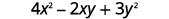简化指数。乘以。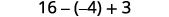减去。添加。31

67

## 将短语翻译成带整数的表达式

##### 示例$$\PageIndex{31}$$

$$\begin{array}{lc} \text{} & \text{the } \textbf{sum } \underline{\text{of}} \; –8 \; \underline{\text{and}} −12 \text{ increased by } 3 \\ \text{Translate.} & [8+(−12)]+3 \\ \text{Simplify. Be careful not to confuse the} \; \; \; \; \; \; \; \; \; \; & (−4)+3 \\ \text{brackets with an absolute value sign.} \\ \text{Add.} & −1 \end{array}$$

##### 示例$$\PageIndex{32}$$

$$(9+(−16))+4;−3$$

##### 示例$$\PageIndex{33}$$

$$(−8+(−12))+7;−13$$

## 在应用程序中使用整数

##### 示例$$\PageIndex{34}$$: How to Solve Application Problems Using Integers##### 在应用程序中使用整数。
1. 阅读问题。 确保所有文字和想法都被理解。
2. 确定我们被要求查找的内容。
3. 写一个短语，提供找到它的信息。
4. 将短语@@ 翻译成表达式。
5. 简化表达式。
6. 用完整的句子@@ 回答问题。

• 用计数器减去整数

## 关键概念

• \begin{align} & −a \text{ means the opposite of the number }a \\ & \text{The notation} −a \text{ is read as “the opposite of }a \text{.”} \end{align}
• 数字的绝对值是它在数字线上距离 0 的距离。

数字 n 的绝对值写成所有数字$$|n|$$$$|n|≥0$$ and。

绝对值始终大于或等于零。

• $\begin{array}{lclc} \text{Parentheses} & () & \text{Braces} & \{ \} \\ \text{Brackets} & [] & \text{Absolute value} & ||\end{array}$
• 减法属性
$$a−b=a+(−b)$$
去一个数字等于将其相反的值相加。
• 用于两个有符号数字的乘法和除法：
同样的迹象 结果
• 两个积极因素 阳性
• 两张底片 阳性
如果信号相同，则结果为阳性。
不同的迹象 结果
• 正面和负面 负面的
• 负面和阳性 负面的
如果符号不同，则结果为负数。
• 乘以$$−1$$

$$−1a=−a$$

将数字乘以得$$−1$$出相反的结果。

• 如何在应用程序中使用整数。
1. 阅读问题。 确保所有文字和想法都被理解
2. 确定我们被要求查找的内容。
3. 写一个短语，提供找到它的信息。
4. 将短语@@ 翻译成表达式。
5. 简化表达式。
6. 用完整的句子@@ 回答问题。